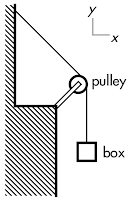20180925

Physics quiz question: rope, pulley, suspended box

Physics 205A Quiz 3, fall semester 2018
Cuesta College, San Luis Obispo, CAA 0.50 kg box is suspended by use of an ideal pulley and rope. Neglect the weights of the pulley and rope. The force with the smallest magnitude is the:
(A) tension force of rope on the box.
(B) weight force of Earth on the box.
(C) net force on the box.
(D) (Not enough information is given.)

Correct answer (highlight to unhide): (C)

The box has two vertical forces acting on it:
Tension force of rope on the box (upwards).
Weight force of Earth on the box (mbox·g = 4.9 N, downwards).
Since the box is stationary, then according to Newton's first law the forces acting on it must sum to a net force of zero, and the tension force must also have a magnitude of 4.9 N. Thus weight and tension force are tied for the having the greatest magnitude, and the net force on the elevator has the smallest magnitude (of zero).

Sections 70854, 70855
Exam code: quiz03pRH5
(A) : 2 students
(B) : 4 students
(C) : 45 students
(D) : 5 students

Success level: 81%
Discrimination index (Aubrecht & Aubrecht, 1983): 0.67